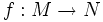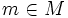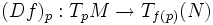# Vertical space

Let$f:M \to N$ be a smooth map of differential manifolds. Given a point$m \in M$, the vertical space at$m$ is the kernel of the map$(Df)_p: T_pM \to T_{f(p)}(N)$.
A vector that lies in the vertical space at$p$ is termed a vertical vector at$p$.